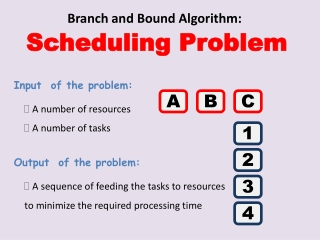Download PresentationBranch and Bound Algorithm:# Branch and Bound Algorithm: - PowerPoint PPT Presentation

Download Presentation##### Branch and Bound Algorithm:

Download Policy: Content on the Website is provided to you AS IS for your information and personal use and may not be sold / licensed / shared on other websites without getting consent from its author. While downloading, if for some reason you are not able to download a presentation, the publisher may have deleted the file from their server.

- - - - - - - - - - - - - - - - - - - - - - - - - - - E N D - - - - - - - - - - - - - - - - - - - - - - - - - - -
##### Presentation Transcript

1. Branch and Bound Algorithm: Scheduling Problem Input of the problem: A B C • A number of resources • A number of tasks Output of the problem: 1 • A sequence of feeding the tasks to resources to minimize the required processing time 2 3 4

2. Different tasks take different time to be processed in each resource A B C 1 2 3 4

3. NP 3 4 2 1 Tasks can be done in any order 3 1 4 2 N! Possible different sequences 3 4 1 2

4. Application 1 Digital processing: Each resource is a processor. All tasks need to pass trough all processors in the fix sequence A,B,C but depending on the task it takes different time for each processor to process them. For example : Processor A: Scanning Processor B: Making a PDF Processor C: Exporting a PDF Task 1: A one page plain text document Task 2: A 10 page document with pictures Task 3: A 5 page html document. Task 4: …

5. Application 2 Production line: Each product (task) need to pass trough all machines (resources) in the production line but, the time depends on what kind of customization the customer has ordered for that production. For example: Machine A: Solding Machine B: Painting Machine C: Packaging Task 1: A black car with airbag Task 2: A red car without airbag with CD player Task 3: A white car with leather seats Task 4: …

6. Decision tree (Brute force)

7. Greedy Algorithm A possible greedy algorithm might start with selecting the fastest tasks for processor A. A B C 1 2 3 : A 4 2 3 1 4

8. Greedy solution T(4,2,3,1) = 34 A B C 1 4 2 3 1 2 2 1 4 3 3 4 2 1 3 4

9. B&B solution T(4,1,2,3) = 26 A B C 1 1 3 4 2 2 1 4 2 3 3 4 1 2 3 4

10. Branch and bound Algorithm Define a bounding criteria for a minimum time required by each branch of the decision tree For level 1: For level 2:

11. Minimum This next Level 1 A B C b(1)= 7+(6+5+4+4)+1=27 b(2)= 5+(6+5+4+4)+1=25 b(3)= 6+(6+5+4+4)+2=27 b(4)= 3+(6+5+4+4)+1=23 1 2 3 4 Bounds: T ≥27 T ≥25 T ≥27 T ≥23

12. Minimum This next Level 2 b(4,1)= (3+7)+(6+5+4)+1=26 b(4,2)= (3+5)+(6+5+4)+1=24 b(4,3)= (3+6)+(6+5+4)+2=26 A B C 1 2 3 4 Bounds: T ≥26 T ≥24 T ≥26

13. Solve the branch 4-2-x-x Actual T(4,2,1,3) = 29 Actual T(4,2,3,1) = 34 A B C 1 Actual Time: T(min)= 29 T ≥26 T ≥24 T ≥26 2 3 Because the actual time T(4,2,1,3) > b(4,1) and T(4,2,1,3) > b(4,3,…), we must solve these branches, too. 4

14. Actual T(4,1,2,3) = 26 Actual T(4,1,3,2) = 28 Actual T(4,3,1,2) = 29 Actual T(4,3,2,1) = 34 A B C 1 2 3 Bounds: T ≥26 T ≥24 T ≥26 4 T(min)= 29 Actual Times: T(min)= 29 T(min)= 26

15. T ≥27 T ≥25 T ≥27 T ≥23 Must be solved The actual T(4,…)=26 is greater than the bound 25 so we must calculate branch T(2,…) A B C 1 2 3 Bounds: Actual Time: 4 T = 26

16. b(2,1)= (5+7)+(6+4+4)+1=27 b(2,3)= (5+6)+(6+4+4)+3=28 b(2,4)= (5+3)+(6+4+4)+1=23 A B C The only candidate that can outperform T(4,1,2,3) is T(2,4,…) so we calculate it: 1 Actual T(2,4,1,3) = 29 Actual T(2,4,3,1) = 34 2 3 4

17. T ≥27 T ≥25 T ≥27 T ≥23 T = 29 T = 26 Bounds greater than 26! So the best time is T(4,1,2,3) and we don’t need to solve the problem for any other branch because we now their minimum time, already. A B C 1 2 3 Bounds: Actual Time: 4

18. Summary • Using only the first level criteria we reduce the problem by 50% (omitting 2 main branches). • Using the second level criteria we can reduce even more.